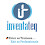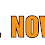### OBJECT ORIENTED ANALYSIS AND DESIGN (OOAD)–Question Bank

Anna University

Department of Computer Science and Engineering

OBJECT ORIENTED ANALYSIS AND DESIGN

QUESTION BANK

Subject Code : CS2353

Subject Name : OBJECT ORIENTED ANALYSIS AND DESIGN (OOAD)

Semester : VI

UNIT-I

PART-A

1. What is Object-Oriented Analysis and Design?

2. Define Design Class Diagrams

3. What is the UML?

4. What are the three ways and perspectives to Apply UML?

5. Define the Inception step?

6. What Artifacts May Start in Inception?

7. What are Actors?

8. What is a scenario?

9. Define Use case.

10. What are Three Kinds of Actors?

11. What Tests Can Help Find Useful Use Cases?

12. What are Use Case Diagrams?

13. What are Activity Diagrams?

14. Define Requirements and mention its types.

15. Describe Agile Principles.

16. What is meant by POS?

PART- B

1. Explain about POS generation systems. 

2. Define Inception. Explain about artifacts of Inception 

3. Briefly explain the different phases Unified process. 

4. Explain about Use-Case Model and its Writing Requirements in Context.

5. List out the components of Object-Oriented Analysis and Design.

6. Draw the use case diagram for the process sale and specify actor, use case and scenario.

7. Explain with an example, how use case modeling is used to describe functional requirements, Identify the actors, scenario and use cases for the example. 

8. With an example explain all the use case relationships. 

UNIT-II

Elaboration

PART- A

1. What is Elaboration?

2. What are the tasks performed in elaboration?

3. What are the key ideas and best practices that will manifest in elaboration?

4. What Artifacts May Start in Elaboration?

5. What are the key ideas for Planning the Next Iteration?

6. What is a Domain Model?

7. How the domain model is illustrated?

8. Why Call a Domain Model a "Visual Dictionary"?

9. What are the elements not suitable in a domain model?

10. What are Conceptual Classes?

11. How to Create a Domain Model?

12. How to Find Conceptual Classes?

13. Mention some Conceptual Class Category.

14. Define Association.

15. Why Should We Avoid Adding Many Associations?

16. How to Name an Association in UML?

17. Define Aggregation and composition.

18. What is composition?

19. Mention the guidelines that suggest when to show aggregation.

20. What is an activity diagram?

PART –B

1. Write briefly about elaboration and discuss the differences between Elaboration and Inception

With examples. 

2. Illustrate the concept of Domain model with examples. 

3. Describe the strategies used to identify Conceptual Classes, Describe the steps to create a domain model used for representing conceptual classes.

4. Explain about activity diagram with an example. 

5. Explain about Aggregations and compositions 

6. Explain all attribute specified in the Domain model with example.

7. Explain Domain model refinement with suitable diagram. 

8. Explain to find the conceptual class hierarchies with suitable diagram.

Unit-III

System Sequence Diagrams

PART- A

1. What is the use of System Sequence Diagrams?

2. What is meant by System Behavior?

3. What is meant by Inter-System SSDs?

4. Define System Events and the System Boundary.

5. How to Naming System Events and Operations?

6. What is meant by interaction diagram?

7. What is meant by link?

8. What is meant by Messages?

9. How to create an instance?

10. What is meant by Low Coupling?

11. What is meant by High cohesion?

12. Define Controller.

13. What is meant by CRC card?

14. What is meant by Pure Fabrication?

15.List the relationships used in class diagram.

PART- B

1. Illustrate with an example, the relationship between sequence diagram and use cases.

2. Explain about UML Interaction Diagram Notation? 

3. Design the Model and Creating Design Class Diagrams.

4. What are concepts involved in logical architecture refinement?

5. With suitable diagram specify all the relationship between SSD and use case. 

6. Explain the logical architecture and UML package diagram. 

7. Explain the logical architecture refinement with diagram.

8. Explain with an example Interaction diagram. 

Unit-IV

GRASP

PART- A

1. How to Choosing the Initial Domain Object?

2. How to Connecting the UI Layer to the Domain Layer?

3. Mention the Interface and Domain Layer Responsibilities.

4. What is Design patterns.

5. How to Apply the GRASP Pattern?

6. Define Responsibilities and Methods.

7. Who is creator?

8. List out some scenarios that illustrate varying degrees of functional cohesion.

9. Define Modular Design.

10. What are the advantages of Factory objects?

11. Designing for Non-Functional or Quality Requirements.

12. Abstract for Factory (GoF) for Families of Related Objects.

13. What is meant by Abstract Class Abstract Factory?

14. What is meant by Fine-Grained Classes?

PART- B

1. Explain Grasp: designing objects with responsibilities. 

2. How to Determining the Visibility of the Design Model? 

3. Explain about Patterns for Assigning Responsibilities. 

4. Designing the Use-Case Realizations with GOF Design Patterns. 

5. Write short notes on adapter,singleton,factory and observer patterns.

6. Explain LOW and HIGH cohesion with near diagram. 

7. With neat diagram explain CONTROLLER.

Unit-V

UML state diagrams and modeling

PART- A

1. Define post condition.

2. Define Attributes.

3. What is the use of operation contracts?

4. When Are Contracts Useful?

5. Mention the Guidelines for Contracts.

6. What are Steps for Mapping Designs to Code?

7. Creating Class Definitions from DCDs.

8. What are the Benefits of Iterative Development?

9. Define Events, States, and Transitions.

10. What is meant by State chart Diagrams?

11. Utility of Use Case State chart Diagrams.

12. List out the types of Events.

13. Define External event.

14. Define internal event.

15. Define temporal event.

16.Give the meaning of Event, State and transition.

PART- B

1. Explain UML State Machine Diagrams and Modeling.

2. What is the operation of contracts works with example.

3. Explain about implementation model( Mapping Designs to Code).

4. Discuss about UML Deployment and Component Diagrams. Draw the diagrams for a banking application.

1.2."Insightful" is the perfect word to describe this wonderful writing of yours. The artistic blend of this subject with your tone of writing made this a great read. Much love 😘.
How to bottom

3.Thanks a lot for sharing such a good source with all, i appreciate your efforts taken for the same. I found this worth sharing and must share this with all.

Dot Net Training in Chennai | Dot Net Training in anna nagar | Dot Net Training in omr | Dot Net Training in porur | Dot Net Training in tambaram | Dot Net Training in velachery

4.5.6.7.This blog is very attractive, I am grateful to the holder of this site. I visit this weblog once.

8.